# area of triangle in coordinate geometry

area of triangle in coordinate geometry
Go back to  'Coordinate Geometry'

In this mini-lesson, we are going to learn about the area of a triangle in coordinate geometry and some interesting facts around them.

In Geometry, a triangle is a three-sided polygon that has three edges and three vertices.

The area of the triangle is the space covered by the triangle in a two-dimensional plane.

The formula for the area of a triangle is $$\dfrac{1}{2}\times\text{base}\times\text{altitude}$$.

Please check the visualization of the area of a triangle in coordinate geometry. Enter the values of A, B, C, or drag the vertices of the triangle and see how the area changes for different values.

Let's find out the area of a triangle in coordinate geometry.

## What Is the Area of a Triangle in Coordinate Geometry?

Coordinate geometry is defined as the study of geometry using the coordinate points.
The area of a triangle in coordinate geometry can be calculated if the three vertices of the triangle are given in the coordinate plane.

Let us understand this with an example.

Consider the following three points:

$\begin{array}{l}A = \left( { - 2,\;1} \right)\\B = \left( {3,\;2} \right)\\C = \left( {1,\;5} \right)\end{array}$

If you plot these three points in the plane, you will find that they are non-collinear, which means that they can be the vertices of a triangle, as shown below: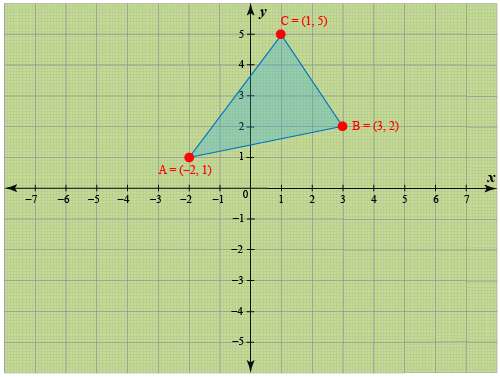Now, with the help of coordinate geometry, we can find the area of this triangle.

Let us learn more about it in the following section.

## How Do You Calculate the Area of A Triangle in Coordinate Geometry?

Note that the area of any triangle is:

${\rm{Area}} = \frac{1}{2}\;bh$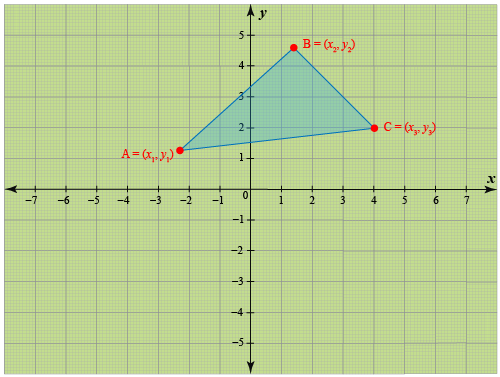If we need to find the area of a triangle coordinates, we use the coordinates of the three vertices.

Observe the following figure carefully.
In this figure, we have drawn perpendiculars AD, CF, and BE from the vertices of the triangle to the horizontal axis.

Notice that three trapeziums are formed: ACFD, BCFE, and ABED.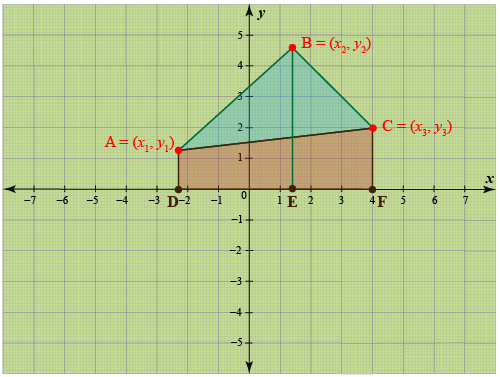We can express the area of a triangle in terms of the areas of these three trapeziums.

${\rm{Area}}\left( {{\rm{\Delta ABC}}} \right){\rm{ = }}\left\{ \begin{array}{l}{\rm{Area}}\;\left( {{\rm{Trap}}{\rm{.}}\;{\rm{ABED}}} \right)\\ \,\,\,\,\,\,\,\,\,\,\,\, \,\,\,\,\,\,\,\,\,\,\,\,\,\,\,\,\,\,\,\,\,\,\,\,\,\,\,\,\,\,\,\,\, + \\{\rm{Area}}\;\left( {{\rm{Trap}}{\rm{.}}\;{\rm{BEFC}}} \right)\\ \,\,\,\,\,\,\,\,\,\,\,\,\,\,\,\,\,\,\,\,\,\,\,\,\,\,\,\,\,\,\,\,\,\,\,\,\,\,\,\,\,\,\,\,\,\, - \\{\rm{Area}}\;\left( {{\rm{Trap}}{\rm{.}}\;{\rm{ACFD}}} \right)\end{array} \right.$

Now, the area of a trapezium in terms of the lengths of the parallel sides (the bases of the trapezium) and the distance between the parallel sides (the height of the trapezium):

${\rm{Trapezium}}{\rm{}}\;{\rm{Area}} = \frac{1}{2} \times \;{\rm{Sum}}\;{\rm{of}}\;{\rm{bases}}\;{\rm{ \times }}\;{\rm{Height}}$

Consider any one trapezium, say ACFD. Its bases are AD and CF, and its height is DF. AD and CF can easily be seen to be the y coordinates of A and C, while DF is the difference between the x coordinates of C and A. Similarly, the bases and heights of the other two trapeziums can be easily calculated. Thus, we have:

\begin{align}&{\rm{Area}}\;\left( {{\rm{Trap}}{\rm{.}}\;{\rm{ACFD}}} \right) = \frac{1}{2} \times \left( {AD + BE} \right) \times DE\\&\qquad\qquad\qquad\qquad\quad= \frac{1}{2} \times \left( {{y_1} + {y_2}} \right) \times \left( {{x_2} - {x_1}} \right)\\&{\rm{Area}}\;\left( {{\rm{Trap}}{\rm{.}}\;{\rm{BEFC}}} \right) = \frac{1}{2} \times \left( {CF + BE} \right) \times FE\\&\qquad\qquad\qquad\qquad\quad= \frac{1}{2} \times\left( {{y_2} + {y_3}} \right) \times \left( {{x_3} - {x_2}} \right)\\&{\rm{Area}}\;\left( {{\rm{Trap}}{\rm{.}}\;{\rm{ABED}}} \right) = \frac{1}{2} \times \left( {AD + CF} \right) \times DF\\&\qquad\qquad\qquad\qquad\quad= \frac{1}{2} \times \left( {{y_1} + {y_3}} \right) \times \left( {{x_3} - {x_1}} \right)\end{align}

This is the expression for the area of the triangle in terms of the coordinates of its vertices.

However, we should try to simplify it so that it is easy to remember.

For that, we simplify the product of the two brackets in each terms:

$\begin{array} &=\dfrac12 ({x_2}{y_1} - {x_1}{y_1} + {x_2}{y_2} - {x_1}{y_2})\\ + \dfrac12({x_3}{y_2} - {x_2}{y_2} + {x_3}{y_3} - {x_2}{y_3})\\ -\dfrac12 ({x_3}{y_1} - {x_1}{y_1} + {x_3}{y_3} - {x_1}{y_3}) \end{array}$

Take the common term $$\dfrac12$$ outside the bracket

$\begin{array} &=\dfrac12({x_2}{y_1} - {x_1}{y_1} + {x_2}{y_2} - {x_1}{y_2}\\ +{x_3}{y_2} - {x_2}{y_2} + {x_3}{y_3} - {x_2}{y_3} \\- {x_3}{y_1} + {x_1}{y_1} - {x_3}{y_3} + {x_1}{y_3}) \end{array}$

Thus,

$\begin{array}{l}{\rm{Area}}\;\left( {\Delta ABC} \right)= \frac{1}{2}\left\{ \begin{array}{l}{x_1}\left( {{y_2} - {y_3}} \right) + {x_2}\left( {{y_3} - {y_1}} \right) + {x_3}\left( {{y_1} - {y_2}} \right)\end{array} \right\}\end{array}$

As the area is always positive

$$\therefore$$$\begin{array}{\rm{Area}}\;\left( {\Delta ABC} \right)= \frac{1}{2}\left| \begin{array}{l}{x_1}\left( {{y_2} - {y_3}} \right) + {x_2}\left( {{y_3} - {y_1}} \right) + {x_3}\left( {{y_1} - {y_2}} \right)\end{array} \right|\end{array}$

This is a symmetric expression, and there is a an easy technique to remember it, which we will now discuss as Determinants Method.

Determinants Method

Write the coordinates as shown below, in the form of a grid with the third row as constant entries:

$\begin{array}{l}{x_1} & & {x_2} & & {x_3}\\{y_1} & & {y_2} & & {y_3}\\1 & & 1 & & 1\end{array}$

Now, the first term in the expression for the area is $${x_1}\left( {{y_2} - {y_3}} \right)$$.

To write this, we ignore the terms in the first row and column other than the first term, and proceed according to the following visual representation (the cross arrows represent multiplication):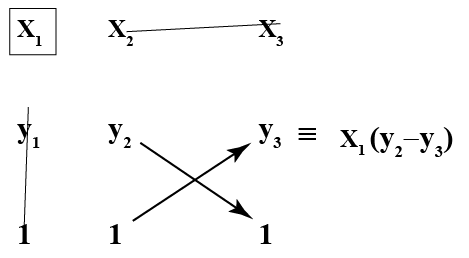The second term in the expression for the area is $${x_2}\left( {{y_3} - {y_1}} \right)$$ .

To write this, we ignore the terms in the first row and second column other than the first term in the second column, but this time we reverse the order, that is, we have $${y_3} - {y_1}$$ instead of $${y_1} - {y_3}$$: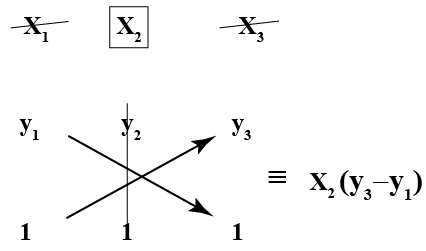Next, the third term in the expression for the area is $${x_3}\left( {{y_1} - {y_2}} \right)$$ .

To write this, we ignore the terms in the first row and third column other than the first term in the third column: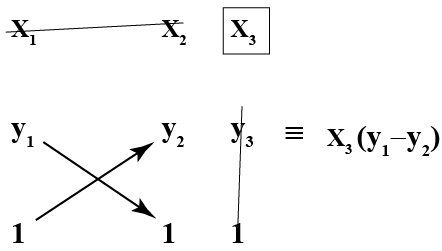Finally, we add these three terms to get the area (and divided by a factor of 2, because we had this factor in the original expression we determined):

$A = \frac{1}{2}\left| \begin{array}{l}{x_1}\left( {{y_2} - {y_3}} \right) + {x_2}\left( {{y_3} - {y_1}} \right)+ {x_3}\left( {{y_1} - {y_2}} \right)\end{array} \right|$

Note that we have put a modulus sign (vertical bars) around our algebraic expression, and removed the negative sign because the area is always positive, we obtained in the original expression.

So even if we get a negative value through the algebraic expression, the modulus sign will ensure that it gets converted to a positive value.

We can write the above expression for area compactly as follows:

$A = \frac{1}{2}\;\left| {\begin{array}{*{20}{c}}{{x_1}}&{{x_2}}&{{x_3}}\\{{y_1}}&{{y_2}}&{{y_3}}\\1&1&1\end{array}} \right|$

## Solved Examples

 Example 1

Noah wants to find the area of this triangle by the determinants method.

Consider a triangle with the following vertices:

$\begin{array}{l}A = \left( { - 1,\;2} \right)\\B = \left( {2,\;3} \right)\\C = \left( {4,\; - 3} \right)\end{array}$

What is the area of this triangle?

Solution: To illustrate, we will calculate each of the three terms in the formula for the area separately, and then put them together to obtain the final value.

$\left| {\begin{array}{*{20}{c}}{ - 1}&2&4\\2&3&{ - 3}\\1&1&1\end{array}} \right|$

First Term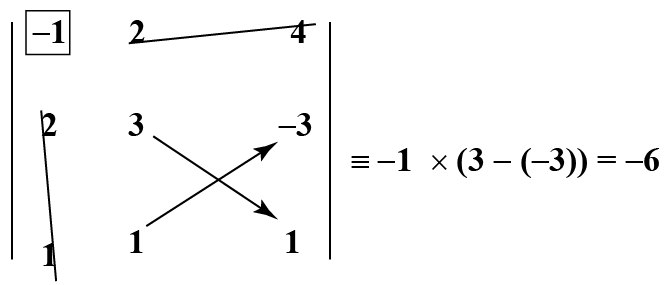Second Term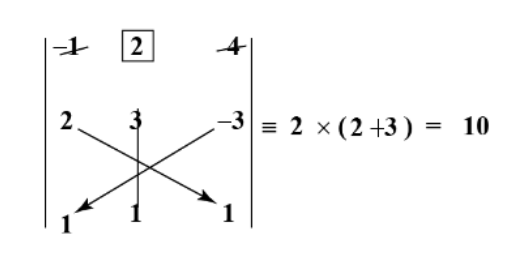Third Term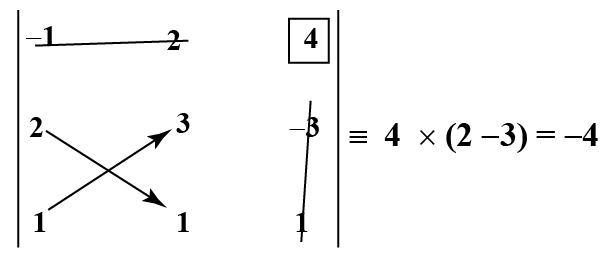Finally, we put these three values together, taking care not to ignore the factor of 2, and also to use the modulus sign to get a positive value:

\begin{align}&{\rm{Area}}\;\left( {\Delta ABC} \right)\\ &= \frac{1}{2}\left| {\left( { - 6} \right) + \left( {10} \right) + \left( { - 4} \right)} \right|\\ &= \frac{1}{2} \times 10\\ &= 5\;{\rm{sq}}{\rm{.}}\;{\rm{units}}\end{align}

 $$\therefore$$ The area of triangle is 5 unit square.
 Example 2

Ethan is unable to find the area of a triangle with the following vertices. an you help him?

$\begin{array}{l}A\left( {3,\;4} \right)B\left( {4,\;7} \right) \text{and C}\left( {6,\; - 3} \right)\end{array}$

Solution

We have:

$\begin{array}{\rm{Area}}\;\left( {\Delta ABC} \right)= \frac{1}{2}\left| \begin{array}{l}{x_1}\left( {{y_2} - {y_3}} \right) + {x_2}\left( {{y_3} - {y_1}} \right) + {x_3}\left( {{y_1} - {y_2}} \right)\end{array} \right|\end{array}$\begin{array}{\rm{Area}}\;\left( {\Delta ABC} \right)= \frac{1}{2}\left| \begin{array}{l}{3}\left( {7 - (-3)} \right) + {4}\left( {(-3) - (-4)} \right) + {6}\left( {4 - (7)} \right)\end{array} \right|\end{array} \\\begin{align}\qquad &= \frac{1}{2}\;\left| {30 + 4 - 18} \right|\, \\&=\frac{1}{2} \times 16 \\&= 8\;{\rm{sq}}{\rm{.}}\;{\rm{units}}\end{align}

 $$\therefore$$  The area of a triangle is 8 unit square.
 Example 3

Find the area of the triangle whose vertices are: $\begin{array}{l}A\left( {1,\;-2} \right)\\B\left( {-3,\;4} \right)\\C\left( {2,\; 3} \right)\end{array}$

Solution

\begin{align}&{\rm{Area}} = \frac{1}{2}\left| {\,\begin{gathered}{}1&3&2\\{-2}&4&{-3}\\1&1&1\end{gathered}\,} \right|\;\begin{gathered}{} \leftarrow &{x\;{\rm{row}}}&{}\\ \leftarrow &{y\;{\rm{row}}}&{}\\ \leftarrow &{{\rm{constant}}}&{}\end{gathered}\\&\qquad= \frac{1}{2}\;\left| \begin{array}{l}1 \times \left( {4 - \left( {-3} \right)} \right) + 3 \times \left( { (-3) -(- 2)} \right)\\ + 2\left( {{-2} - 4} \right)\end{array} \right|\\&\qquad = \frac{1}{2}\;\left| {7 -3 - 12} \right|\, = \frac{1}{2} \times 8 = 4\;{\rm{sq}}{\rm{.}}\;{\rm{units}}\end{align}

 $$\therefore$$  The area of a triangle is 4 unit square.Challenging Questions
• If the distance between the points (2, 3) and (1, q) is 5, find the values of q.
• What is the formula for the area of quadrilateral in coordinate geometry?

## Interactive Questions

Here are a few activities for you to practice.

Select/Type your answer and click the "Check Answer" button to see the result.

## Let’s Summarize

This mini-lesson was aimed at helping you learn about the area of a triangle in coordinate geometry and its characteristics. Hope you enjoyed learning about them and exploring various questions on the area of a triangle in coordinate geometry.

## About Cuemath

At Cuemath, our team of math experts is dedicated to making learning fun for our favorite readers, the students!

Through an interactive and engaging learning-teaching-learning approach, the teachers explore all angles of a topic.

Be it problems, online classes, doubt sessions, or any other form of relation, it’s the logical thinking and smart learning approach that we, at Cuemath, believe in.

## 1. What are the coordinates of the vertices of a triangle?

The coordinates of the vertices of a triangle are $$(x_1,y_1), (x_2,y_2), and (x_3,y_3)$$.

## 2. How do you find the length of a triangle using coordinates?

The distance formula is used to find the length of a triangle using coordinates.

## 3. What is the formula of the area of a triangle in coordinate geometry?

The formula of area of triangle formula in coordinate geometry the area of triangle in coordinate geometry is: $A = \frac{1}{2}\left| \begin{array}{l}{x_1}\left( {{y_2} - {y_3}} \right) + {x_2}\left( {{y_3} - {y_1}} \right)+ {x_3}\left( {{y_1} - {y_2}} \right)\end{array} \right|$

## 4.How do you find the area and perimeter of a triangle with coordinates?

For the area and perimeter of a triangle with coordinates first, we have to find the distance between each pair of points by distance formula and then we apply the formula for area and perimeter.

## 5. How do you find the area of a triangle with 3 coordinates?

Area of triangle with 3 points is: $A = \frac{1}{2}\left| \begin{array}{l}{x_1}\left( {{y_2} - {y_3}} \right) + {x_2}\left( {{y_3} - {y_1}} \right)+ {x_3}\left( {{y_1} - {y_2}} \right)\end{array} \right|$

## 6. How do you find the area of triangle using vertices?

The formula of the area of triangle in coordinate geometry is: $A = \frac{1}{2}\left| \begin{array}{l}{x_1}\left( {{y_2} - {y_3}} \right) + {x_2}\left( {{y_3} - {y_1}} \right)+ {x_3}\left( {{y_1} - {y_2}} \right)\end{array} \right|$

## 7. How do you find the coordinates of an isosceles triangle?

First, we use the distance formula to calculate the length of each side of the triangle. If two sides are equal then it's an isosceles triangle.

## 8. How do you find the coordinates of a right-angled triangle?

First, we use the distance formula to calculate the length of each side of the triangle. If the squares of the smaller two distances equal to the square of the largest distance, then these points are the vertices of a right triangle. or we can use Pythagoras theorem.

## 9.How do you calculate the area of a triangle on a graph?

The area of a triangle on a graph is calculated by the formula of area which is: $A = \frac{1}{2}\left| \begin{array}{l}{x_1}\left( {{y_2} - {y_3}} \right) + {x_2}\left( {{y_3} - {y_1}} \right)+ {x_3}\left( {{y_1} - {y_2}} \right)\end{array} \right|$

## 10. How do you find the missing coordinate of a right angled triangle?

We use the distance formula to calculate the missing coordinate of a right-angled triangle.

More Important Topics
Numbers
Algebra
Geometry
Measurement
Money
Data
Trigonometry
Calculus